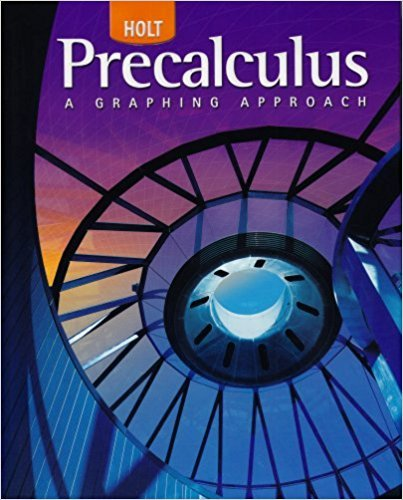×
×

# Solutions for Chapter 9.1: Identities and Proofs## Full solutions for Precalculus | 1st Edition

ISBN: 9780030416477Solutions for Chapter 9.1: Identities and Proofs

Solutions for Chapter 9.1
4 5 0 400 Reviews
23
5
##### ISBN: 9780030416477

This textbook survival guide was created for the textbook: Precalculus, edition: 1. Chapter 9.1: Identities and Proofs includes 66 full step-by-step solutions. This expansive textbook survival guide covers the following chapters and their solutions. Since 66 problems in chapter 9.1: Identities and Proofs have been answered, more than 26154 students have viewed full step-by-step solutions from this chapter. Precalculus was written by and is associated to the ISBN: 9780030416477.

Key Calculus Terms and definitions covered in this textbook
• Algebraic model

An equation that relates variable quantities associated with phenomena being studied

• Boundary

The set of points on the “edge” of a region

• Conjugate axis of a hyperbola

The line segment of length 2b that is perpendicular to the focal axis and has the center of the hyperbola as its midpoint

• Divisor of a polynomial

See Division algorithm for polynomials.

• Finite series

Sum of a finite number of terms.

• Graph of an equation in x and y

The set of all points in the coordinate plane corresponding to the pairs x, y that are solutions of the equation.

• Logarithmic re-expression of data

Transformation of a data set involving the natural logarithm: exponential regression, natural logarithmic regression, power regression

• n-set

A set of n objects.

• Newton’s law of cooling

T1t2 = Tm + 1T0 - Tm2e-kt

• Normal distribution

A distribution of data shaped like the normal curve.

• Partial sums

See Sequence of partial sums.

• Pole

See Polar coordinate system.

• Probability distribution

The collection of probabilities of outcomes in a sample space assigned by a probability function.

• Product of complex numbers

(a + bi)(c + di) = (ac - bd) + (ad + bc)i

The formula x = -b 2b2 - 4ac2a used to solve ax 2 + bx + c = 0.

• Reference angle

See Reference triangle

• Richter scale

A logarithmic scale used in measuring the intensity of an earthquake.

• Tangent

The function y = tan x

• Terminal point

See Arrow.

• yz-plane

The points (0, y, z) in Cartesian space.

×